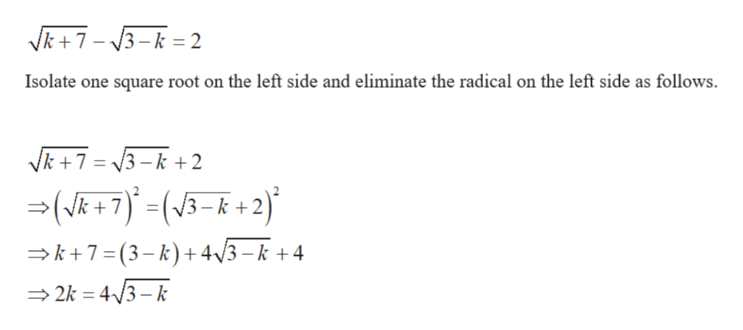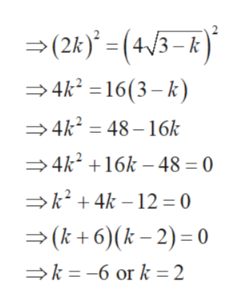square root of (k + 7) - square root of (3 - k) = 2k = ?

Question

square root of (k + 7) - square root of (3 - k) = 2

k = ?

Step 1

The given condition ishelp_outlineImage TranscriptioncloseVk7 3-k= 2 Isolate one square root on the left side and eliminate the radical on the left side as follows. Vk7 3-k+2 (R 7) +2) V3- k7 (3-k) +4/3-k +4 2k 43- k fullscreen
Step 2

Isolate the remaining square root and e...help_outlineImage Transcriptionclose(2k (4/3-k) 4k2 =16(3-k) 4k2=48-16k 4k216k -48 = 0 k24k12 0 (k +6)(k-2) 0 k-6 or k 2 fullscreen

Want to see the full answer?

See Solution

Want to see this answer and more?

Our solutions are written by experts, many with advanced degrees, and available 24/7

See Solution
Tagged in

Algebra